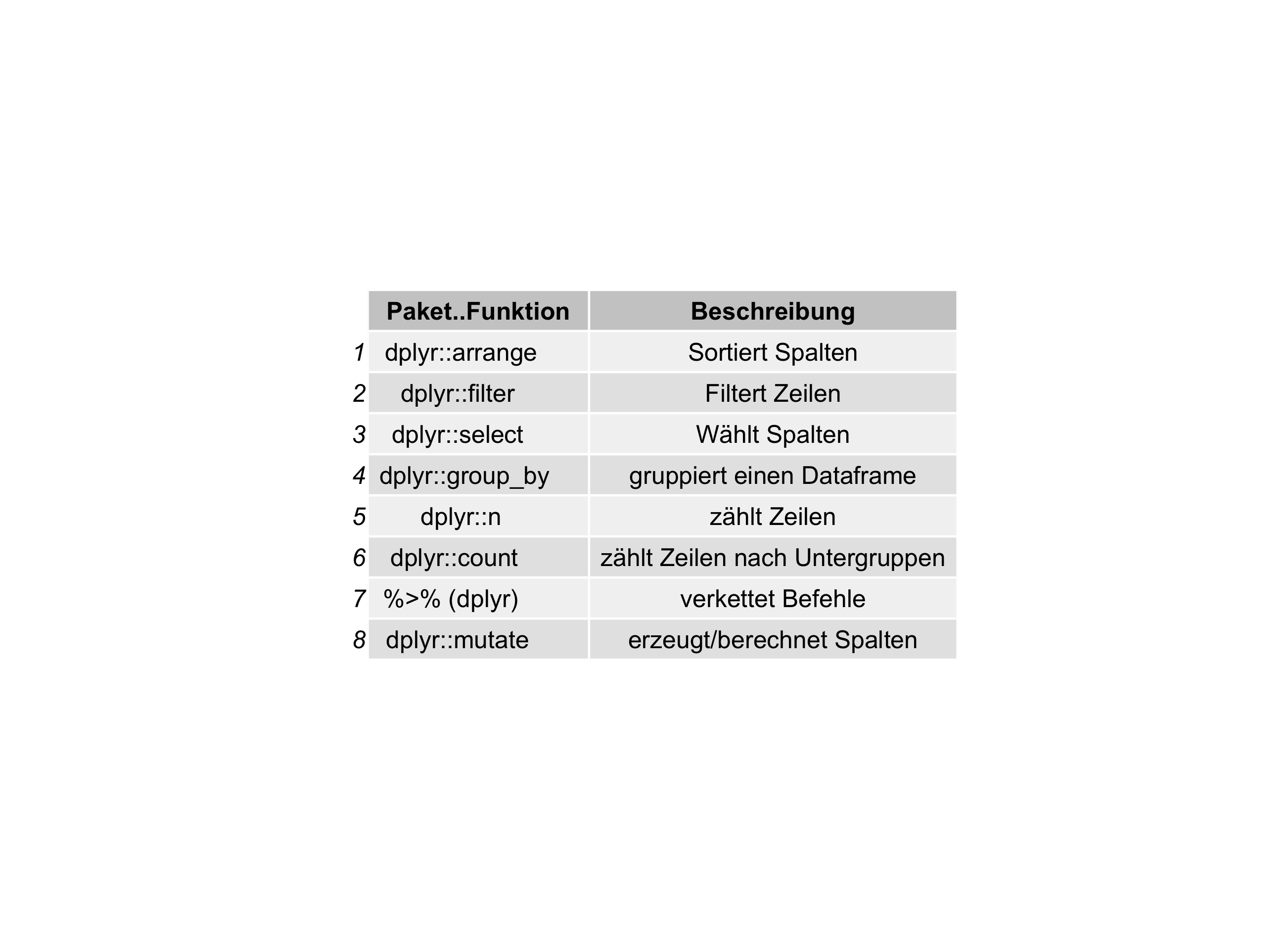tl;dr

Use this convenience function to print a dataframe as a png-plot: tab2grob().

Source the function here: https://sebastiansauer.github.io/Rcode/tab2grob.R

Easiest way in R:

source("https://sebastiansauer.github.io/Rcode/tab2grob.R")


Printing csv-dataframes as ggplot plots

Recently, I wanted to print dataframes not as normal tables, but as a png-plot. See:Why? Well, basically as a convenience function for colleagues who are not into using Markdown & friends. As I am preparing some stats stuff (see my new open access course material here) using RMarkdown, I wanted to prepare the materials ready for using in Powerpoint.

I found out that tables are difficult to copy-paste into Powerpoint. That’s why I thought it may help to print to tables as plots.

So I came up with some function who does this job:

1. Scan a folder for all csv files
2. parse each csv and for each csv file
3. print it as a plot
4. save it as a png

Rcode

tab2plot <- function(path = "") {

# Print csv-dataframes as plots using ggplot2 - for easier handling in Powerpoint & friends
# Arguments
# path: are the csv-files in a subdirectory? Then specify here. Defaults to "" (working directory)
# Value:
# None. Saves each csv-file as png file of the table.

library(tidyverse)
library(stringr)
library(gridExtra)
library(grid)

df <- data_frame(
file_name = list.files(path = path, pattern = "\\w+.csv"),
title = str_extract(file_name, pattern = "\\w+")
)

tt <- ttheme_default(core=list(fg_params=list(hjust=0, x=0.1)))

for (i in seq_along(df$file_name)) { cat(paste0(df$file_name[i], "\n"))
csv_i <- read.csv(paste0(path, df$file_name[i])) #csv_i <- read.csv("includes/Befehle_Cluster.csv") csv_i %>% rownames_to_column %>% dplyr::select(-rowname) -> csv_i p <- arrangeGrob(tableGrob(csv_i, theme = tt)) ggsave(file = paste0("images/Tabellen/","Tabelle_",df$title[i],".png"),  p)
}

}